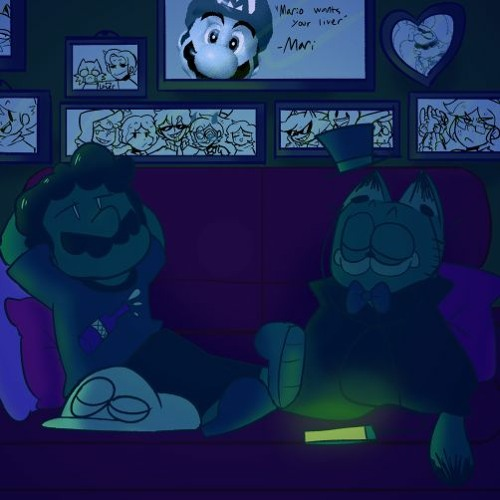# Lo-Fi Hip Hop - Beat to Relax/Shitpost to - Game Over

## FireMF ♬

Lofi LOL
=+=+=+=+=+=+=+=+=+=+=+=+=+=+=+=+=+=+=+=+=+=+
Thumbnail Art by: sketchy-entertainer.tumblr.com